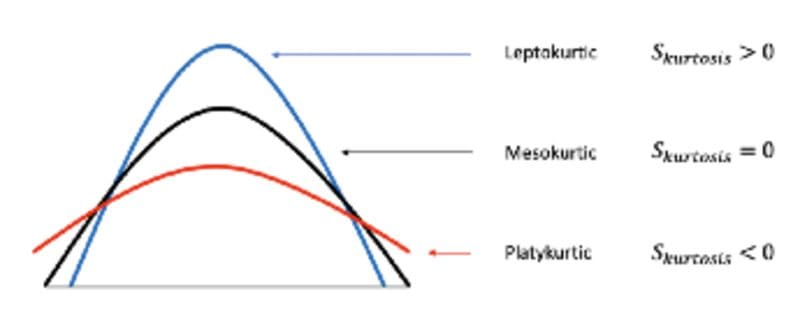# The 8 Basic Statistics Concepts for Data Science￼

Understanding the fundamentals of statistics is a core capability for becoming a Data Scientist. Review these essential ideas that will be pervasive in your work and raise your expertise in the field.

Statistics is a form of mathematical analysis that uses quantified models and representations for a given set of experimental data or real-life studies. The main advantage of statistics is that information is presented in an easy way. Recently, I reviewed all the statistics materials and organized the 8 basic statistics concepts for becoming a data scientist!

• Understand the Type of Analytics
• Probability
• Central Tendency
• Variability
• Relationship Between Variables
• Probability Distribution
• Hypothesis Testing and Statistical Significance
• Regression

# Understand the Type of Analytics

Descriptive Analytics tells us what happened in the past and helps a business understand how it is performing by providing context to help stakeholders interpret information.

Diagnostic Analytics takes descriptive data a step further and helps you understand why something happened in the past.

Predictive Analytics predicts what is most likely to happen in the future and provides companies with actionable insights based on the information.

Prescriptive Analytics provides recommendations regarding actions that will take advantage of the predictions and guide the possible actions toward a solution.

# Probability

Probability is the measure of the likelihood that an event will occur in a Random Experiment.

Complement: P(A) + P(A’) = 1

Intersection: P(A∩B) = P(A)P(B)

Union: P(A∪B) = P(A) + P(B) − P(A∩B)Intersection and Union.

Conditional Probability: P(A|B) is a measure of the probability of one event occurring with some relationship to one or more other events. P(A|B)=P(A∩B)/P(B), when P(B)>0.
Independent Events: Two events are independent if the occurrence of one does not affect the probability of occurrence of the other. P(A∩B)=P(A)P(B) where P(A) != 0 and P(B) != 0 , P(A|B)=P(A), P(B|A)=P(B)

Mutually Exclusive Events: Two events are mutually exclusive if they cannot both occur at the same time. P(A∩B)=0 and P(A∪B)=P(A)+P(B).

Bayes’ Theorem describes the probability of an event based on prior knowledge of conditions that might be related to the event.Bayes’ Theorem.

# Central Tendency

Mean: The average of the dataset.

Median: The middle value of an ordered dataset.

Mode: The most frequent value in the dataset. If the data have multiple values that occurred the most frequently, we have a multimodal distribution.

Skewness: A measure of symmetry.

Kurtosis: A measure of whether the data are heavy-tailed or light-tailed relative to a normal distributionSkewness.Kurtosis.

# Variability

Range: The difference between the highest and lowest value in the dataset.

Percentiles, Quartiles and Interquartile Range (IQR)

• Percentiles — A measure that indicates the value below which a given percentage of observations in a group of observations falls.
• Quantiles— Values that divide the number of data points into four more or less equal parts, or quarters.
• Interquartile Range (IQR)— A measure of statistical dispersion and variability based on dividing a data set into quartiles. IQR = Q3 − Q1Percentiles, Quartiles and Interquartile Range (IQR).

Variance: The average squared difference of the values from the mean to measure how spread out a set of data is relative to mean.
Standard Deviation: The standard difference between each data point and the mean and the square root of variance.Population and Sample Variance and Standard Deviation.

Standard Error (SE): An estimate of the standard deviation of the sampling distribution.Population and Sample Standard Error.

# Relationship Between Variables

Causality: Relationship between two events where one event is affected by the other.

Covariance: A quantitative measure of the joint variability between two or more variables.

Correlation: Measure the relationship between two variables and ranges from -1 to 1, the normalized version of covariance.Covariance and Correlation.

# Probability Distributions

## Probability Distribution Functions

Probability Mass Function (PMF): A function that gives the probability that a discrete random variable is exactly equal to some value.

Probability Density Function (PDF): A function for continuous data where the value at any given sample can be interpreted as providing a relative likelihood that the value of the random variable would equal that sample.

Cumulative Density Function (CDF): A function that gives the probability that a random variable is less than or equal to a certain value.Comparison between PMF, PDF, and CDF.

## Continuous Probability Distribution

Uniform Distribution: Also called a rectangular distribution, is a probability distribution where all outcomes are equally likely.

Normal/Gaussian Distribution: The curve of the distribution is bell-shaped and symmetrical and is related to the Central Limit Theorem that the sampling distribution of the sample means approaches a normal distribution as the sample size gets larger.

Exponential Distribution: A probability distribution of the time between the events in a Poisson point process.

Chi-Square Distribution: The distribution of the sum of squared standard normal deviates.

## Discrete Probability Distribution

Bernoulli Distribution: The distribution of a random variable which takes a single trial and only 2 possible outcomes, namely 1(success) with probability p, and 0(failure) with probability (1-p).

Binomial Distribution: The distribution of the number of successes in a sequence of n independent experiments, and each with only 2 possible outcomes, namely 1(success) with probability p, and 0(failure) with probability (1-p).

Poisson Distribution: The distribution that expresses the probability of a given number of events k occurring in a fixed interval of time if these events occur with a known constant average rate λ and independently of the time.

# Null and Alternative Hypothesis

Null Hypothesis: A general statement that there is no relationship between two measured phenomena or no association among groups. Alternative Hypothesis: Be contrary to the null hypothesis.

In statistical hypothesis testing, a type I error is the rejection of a true null hypothesis, while a type II error is the non-rejection of a false null hypothesis.

Original Source